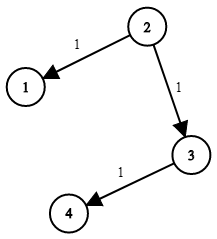엔지니어 게시판
LeetCode 솔루션 분류

# [5/14] 43. Network Delay Time

• mingki 작성
• 작성일

• 247 조회
• 2 댓글

### 본문

[LeetCode 시즌 3] 2022년 5월 14일 문제입니다.

743. Network Delay Time
Medium

You are given a network of `n` nodes, labeled from `1` to `n`. You are also given `times`, a list of travel times as directed edges `times[i] = (ui, vi, wi)`, where `ui` is the source node, `vi` is the target node, and `wi` is the time it takes for a signal to travel from source to target.

We will send a signal from a given node `k`. Return the time it takes for all the `n` nodes to receive the signal. If it is impossible for all the `n` nodes to receive the signal, return `-1`.

Example 1:```Input: times = [[2,1,1],[2,3,1],[3,4,1]], n = 4, k = 2
Output: 2
```

Example 2:

```Input: times = [[1,2,1]], n = 2, k = 1
Output: 1
```

Example 3:

```Input: times = [[1,2,1]], n = 2, k = 2
Output: -1
```

Constraints:

• `1 <= k <= n <= 100`
• `1 <= times.length <= 6000`
• `times[i].length == 3`
• `1 <= ui, vi <= n`
• `ui != vi`
• `0 <= wi <= 100`
• All the pairs `(ui, vi)` are unique. (i.e., no multiple edges.)

댓글 2

## austin님의 댓글

• austin
• 작성일
``````class Solution {
public:
int networkDelayTime(vector<vector<int>>& times, int n, int k) {
int ret = 0;
typedef tuple<int, int> edge;
unordered_set<int> s;
priority_queue<edge, vector<edge>, greater<edge>> q;
vector<vector<edge>> v(n+1);
for(auto &t : times) v[t].emplace_back(t, t);
q.emplace(0, k);
while(s.size() != n && !q.empty()) {
auto [time, node] = q.top();
q.pop();
if (s.find(node) != s.end()) continue;
ret = time;
s.emplace(node);
for(auto [delta, next_node] : v[node]) if (s.find(next_node) == s.end()) q.emplace(time + delta, next_node);
}
return s.size() == n ? ret : -1;
}
};``````

## mingki님의 댓글

• mingki
• 작성일
C++
Runtime: 173 ms, faster than 45.79% of C++ online submissions for Network Delay Time.
Memory Usage: 48.7 MB, less than 11.84% of C++ online submissions for Network Delay Time.
``````class Solution {
public:
int networkDelayTime(vector<vector<int>>& times, int n, int k) {
vector<int> visited(n + 1, 1e9);
vector<vector<vector<int>>> edges(n + 1);
stack<int> st;

for (auto &time : times) {
edges[time].push_back({time, time});
}

st.push(k);
visited[k] = 0;
while (!st.empty()) {
int curr = st.top(); st.pop();
for (auto &edge : edges[curr]) {
if (visited[edge] > visited[curr] + edge) {
visited[edge] = visited[curr] + edge;
st.push(edge);
}
}
}
int result = 0;
for (int i = 1; i < visited.size(); ++i) {
result = max(result, visited[i]);
}
return result == 1e9 ? -1 : result;
}
};``````
전체 85 / 1 페이지

• 등록일 05.21
• 등록일 05.21
• 등록일 05.21
• 등록일 05.21
• 등록일 05.21
• 등록일 05.21
• 등록일 05.21
• 등록일 05.21
• 등록일 05.21

### 새댓글

• 등록자 뚝배기 등록일 05.21
• 등록자 coderoncruise 등록일 05.21
• 등록자 coderoncruise 등록일 05.21
• 등록자 Connie 등록일 05.21
• 등록자 JakeMinSVK 등록일 05.21
• 등록자 JakeMinSVK 등록일 05.21
• 등록자 Connie 등록일 05.20
• 등록자 Jack 등록일 05.20
• 등록자 Connie 등록일 05.19
• 등록자 Connie 등록일 05.19

### Stats

• 현재 접속자 81 명
• 오늘 방문자 132 명
• 어제 방문자 938 명
• 최대 방문자 1,221 명
• 전체 회원수 216 명